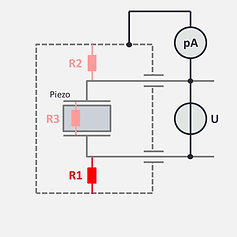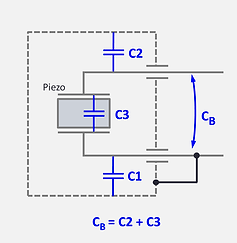top of pagePiezo Accelerometer Tutorial

Accelerometer Properties

# Thermal and Electrical Properties

## Thermal Properties

After the properties in the "sensitivity-axis" and the "frequency-axis" the third domain of utmost importance is the temperature. Regarding the temperature we can distinguish between the ability to withstand temperature and the temperature response.

### Temperature Capability

The capability to survive a maximal (but also a minimal) temperature is given in first line by the piezo material. We have seen a few examples in the chapter about the piezoelectric effect. Most of the time it is the Curie temperature of the piezo material which determines the temperature capability. Thereby we must always keep a respect distance from the Curie temperature!
Of course all the other parts of the sensor must also be capable to maintain their function at the extreme temperatures and finally also the packaging method used by the sensor manufacturer is very important. The absolute maximal (or minimal) temperature that the accelerometer may be exposed to is normally called survival temperature or short term survival temperature. These terms a bit vague and not standardised. For the exact meaning one should refer to the manufacturer's definition, but it implies that the sensor can be exposed to these temperatures without suffering permanent damage or degradation of performance.
Without additional specification, it must be assumed that the sensor is exposed to these temperatures entirely but we sometimes find separate requirements for the «unit mounting temperature» (UMT) and the «surrounding air temperature» (SAT)

### Operational Temperature Range

Similar to the dynamic range we may define a temperature range from the lowest to the highest operational temperature limits within which the specified characteristics of the accelerometer are guaranteed. Besides a certain variation of the sensitivity (which we call temperature response) the most important parameter, influenced by temperature, is the internal resistance of the piezo element. For certain materials the reduction of the resistance is more limiting than the Curie temperature.
As we have seen the internal resistance has a great influence on the frequency response at low frequencies. Therefore this aspect has to be considered when low frequency measurement at higher temperatures is required.
The restrictions due to the internal resistance and the Curie temperature influence the upper limit or the operational temperature range. However sometimes there is also a limit on the low side. Some piezo materials undergo a phase transition and will no longer function below a certain temperature. Fortunately, this effect is reversible.

Piezoelectric accelerometers may also exhibit a more or less pronounced  reaction to temperature variations. These phonemes are addressed in the chapter “Disturbing Factors".

### Temperature Response (temperature sensitivity error)

The temperature response indicates the error of the sensitivity at temperature with respect to room temperature.

It is normally expressed in per cent, where 100% is the sensitivity at room temperature.
For example, a typical statement might be
± 5 % from 20°C to 200°C and ± 8 % from 20°C to -50°CTypical temperature response curve and related specification.

#### Measuring Setup

To carry out a temperature response measurement we need an extension from the shaker into an oven in order to heat up the unit under test without heating the reference sensor. If necessary we can use a cooling device. As stimulation we use a sinusoidal vibration input at the same level and frequency as for the calibration test.
Mechanically we have to verify that there is no axial nor transversal resonance near the test frequency, that could influence the vibration level from one end to the other over the extension rod.
Thermally we have to consider the delay of the temperature to heat up the UUT. We can either make discrete temperature steps and take different measurements after letting the transducer warm up, or we can increase the temperature very slowly and take a continuous measurement.
As result we plot the sensitivity error in percent (with respect to the reading at room temperature) versus temperature.Oven

Sinusoidal vibration input

Reference
transducer

Cooling

Unit under test

Test set-up for the temperature response measurement

#### Influencing design elements

The maximum temperature is clearly determined by the piezo material, and the variation of sensitivity and resistance with temperature also depends almost exclusively on it.

The packaging method is often of great importance for the behaviour of the resistance at temperature.

Of course, the other materials and joining techniques used must also be suitable for the operating conditions.

#### Charge Mode vs. Voltage Mode

If a piezoelectric sensor is used in voltage mode, the temperature response of the sensor is generally not the same as in charge mode!

Temperature Response
Temperature Capability
Electrical Characteristics

## Electrical Characteristics

The main electrical characteristics of a piezoelecric accelerometer are the internal resistance and the capacitance besides the output signal itself.

### Sinal Output: Charge vs Voltage

We consider here only the pure charge output accelerometer, which has no integrated electronics. The accelerometer with charge output should always be operated with a dedicated charge converter as already outlined in this tutorial.

For more detailed information please refer to the chapter "Signal Conditioners".

If for some reason the voltage at the output is of interest, care must be taken that any additional capacitance connected to the accelerometer will reduce the reading of the voltage U.
The charge is Q=U·C , where C  is the total capacitance.
The total capacitance is the sum of the output capacitance of the accelerometer, the connecting cable and the input capacitance of the voltmeter.

### Resistance

We have seen before that the internal resistances of a piezoelectric accelerometer have agreat influence to the correct functioning and are therefore important.

In a symmetric accelerometer we can find three individual resistances:

R1 between pole 1 and the casing,

R2 between pole 2 and the casing,

R3 between pole 1 and pole 2

However the three resistances cannot be accessed for a simple measurement because they are always wired together in a delta connection. We may call the three "corresponding" resitances Rx, Ry and Rz.Individual resistances R1, R2 and R3 versus resistances Rx, Ry and Rz wired in a delta connection

Now the resistors Rx, Ry and Rz are exactly the ones that are important for the function with external electronics. If for example Rz is low, it doesn't matter if it's because of a low R3 or because R1 and R2 are both low.

When we talk about the internal resistance of an accelerometer we mean always the combined resistance Rz. The same applies to the pole to case resistances Rx and Ry.

#### Measurement of Rx, Ry and Rz

The resistances of the accelerometer are very high (in a healthy state). This means that we use a Megohmmeter as it is commonly used to measure an insulation resistance. The recommended voltage is 50 V or 100V. However, care must be taken if a measurement is to be made at temperature. In this case it is possible that the polarisation of the piezo element is affected and therefore a standard multimeter with a few volts test voltage is the better solution.

Sometimes (for example for trouble shooting) the effective values of the resistances R1, R2 or R3 are required. To do that it is recommended to use the 4 wire method, as indicated below.

#### Measurement of R1, R2 and R3 (4 wire method)

​The real values for R1, R2 and R3 can be determined directly using a 4 wire method with separate voltage supply and pico-Ampere meter  as per the sketches below.

This method assumes that the values of R1, R2 and R3 are substantially higher than the internal resistances of the pA meter and the voltage source (which should normally be the case).

The resistance is in each case R=U/iDiagrams for the measurement of the individual resistances R1, R2 and R3 using the 4 wire method

It is recommended to start the measurements with R3 and then R1 and R2 because the discharge of the piezo element (time constant R3C3) after measurement of R1 and R2 might disturb the measurement of R3 .

### Capacitance

​The internal capacitance has practically no significance for the good function of a piezoelectric accelerometer in connection with a charge converter. In contrast to this, the pole to case capacities of a symmetrical accelerometer are important. They should as small as possible and especially equal.

More explanations are found in the chapter "Signal Conditioners".

Similar to the resistances we can find three individual capacities in a symmetric accelerometer:

C1 between pole 1 and the casing,

C2 between pole 2 and the casing,

C3 between pole 1 and pole 2

Again the the capacities cannot be accessed directly because they are wired together in a delta connection.

The three corresponding capacities Cx, Cy and Cz that are accessible to measure from outside are also the ones to be considered for the function with external electronics.Individual Capacities C1, C2 and C3 versus Cx, Cy and Cz wired in a delta connection

By internal capacity of an accelerometer we always mean the combined capacity Cz.

The pole to case capacities are Cx and Cy.

#### Measurement

Any LCR meter or capacitance meter can be used to carry out the measurement of the combined pole to case capacities Cx, Cy or the internal capacity Cz. When measuring small capacitance values, make sure that parasitic capacitances caused by the connection wiring and the measurement setup are avoided.

If for some reason the individual values of the capacitances C1, C2 and C3 are needed, the measurements can be made using the short-circuit bridges according to the sketches below.Diagrams for the measurement of the individual capacities C1, C2 and C3 using short circuit bridges.

With  CA, CB and CC the individual capacities can be calculated as shown below, however for a correct result the resolution of the highest value of CA, CB and CC must be sufficient compared with the significant digits of the lowest value.Resistance
Capacitance

This is the continuation of the green and the yellow path

bottom of page